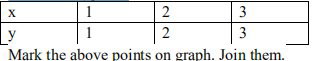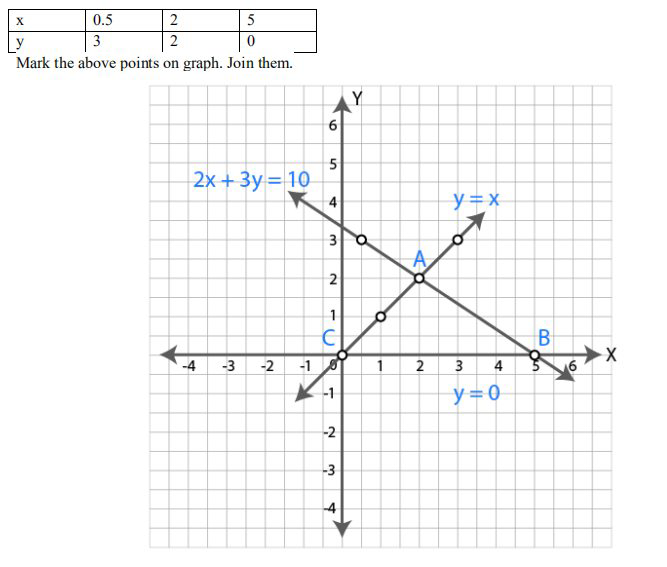# ML Aggarwal Solutions Class 9 Mathematics Solutions for Coordinate Geometry Exercise 19.3 in Chapter 19 - Coordinate Geometry

Question 13 Coordinate Geometry Exercise 19.3

. Find graphically the co-ordinates of the vertices of the triangle formed by the lines y = 0, y = x and 2x +

3y= 10. Hence find the area of the triangle formed by these lines.

y = 0 ..(i)

The graph of y = 0 is the X axis.

y = x ..(ii)

When x = 1, y = 1.

When x = 2, y = 2.

When x = 3, y = 3.2x+3y = 10 ..(iii)

3y = 10-2x

y = (10-2x)/3

When x = 0.5, y = (10-2×0.5)/3 = (10-1)/3 = 9/3 = 3

When x = 2, y = (10-2×2)/3 = (10-4)/3 = 6/3 = 2

When x = 5, y = (10-2×5)/3 = (10-10)/3 = 0From the graph, it is clear that the vertices of the triangle formed by the lines are A(2,2), B(5,0) and C(0,0).

Area of triangle formed by these lines = ½ ×base×height

= ½ ×5×2

= 5 sq. units

Hence area of the triangle is 5 sq. units.

Related Questions

Lido

Courses

Teachers

Book a Demo with us

Syllabus

Maths
CBSE
Maths
ICSE
Science
CBSE

Science
ICSE
English
CBSE
English
ICSE
Coding

Terms & Policies

Selina Question Bank

Maths
Physics
Biology

Allied Question Bank

Chemistry
Connect with us on social media!